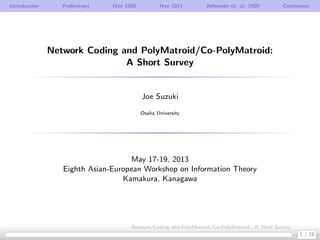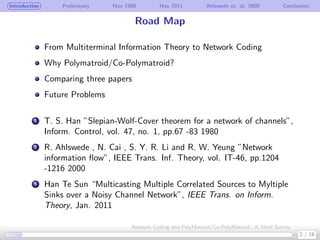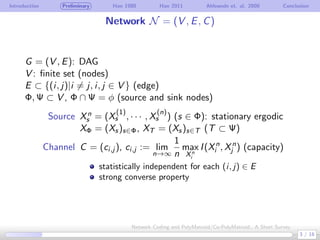Successfully reported this slideshow.

# Eighth Asian-European Workshop on Information Theory: Fundamental Concepts in Information Theory

1

Share×
1 of 16
1 of 16

# Eighth Asian-European Workshop on Information Theory: Fundamental Concepts in Information Theory

1

Share

Kamakura, Kanagawa, JAPAN
May 17-19, 2013
Editors: Hiroyoshi Morita
A. J. Han Vinck
Te Sun Han

Kamakura, Kanagawa, JAPAN
May 17-19, 2013
Editors: Hiroyoshi Morita
A. J. Han Vinck
Te Sun Han

## More Related Content

### Related Books

Free with a 14 day trial from Scribd

See all

### Related Audiobooks

Free with a 14 day trial from Scribd

See all

### Eighth Asian-European Workshop on Information Theory: Fundamental Concepts in Information Theory

1. 1. Introduction Preliminary Han 1980 Han 2011 Ahlswede et. al. 2000 Conclusion Network Coding and PolyMatroid/Co-PolyMatroid: A Short Survey Joe Suzuki Osaka University May 17-19, 2013 Eighth Asian-European Workshop on Information Theory Kamakura, Kanagawa 1 / 16 Network Coding and PolyMatroid/Co-PolyMatroid:, A Short Survey
2. 2. Introduction Preliminary Han 1980 Han 2011 Ahlswede et. al. 2000 Conclusion Road Map From Multiterminal Information Theory to Network Coding Why Polymatroid/Co-Polymatroid? Comparing three papers Future Problems . 1 T. S. Han ”Slepian-Wolf-Cover theorem for a network of channels”, Inform. Control, vol. 47, no. 1, pp.67 -83 1980 . 2 R. Ahlswede , N. Cai , S. Y. R. Li and R. W. Yeung ”Network information ﬂow”, IEEE Trans. Inf. Theory, vol. IT-46, pp.1204 -1216 2000 3 Han Te Sun “Multicasting Multiple Correlated Sources to Myltiple Sinks over a Noisy Channel Network”, IEEE Trans. on Inform. Theory, Jan. 2011 2 / 16 Network Coding and PolyMatroid/Co-PolyMatroid:, A Short Survey
3. 3. Introduction Preliminary Han 1980 Han 2011 Ahlswede et. al. 2000 Conclusion Network N = (V , E, C) G = (V , E): DAG V : ﬁnite set (nodes) E ⊂ {(i, j)|i ̸= j, i, j ∈ V } (edge) Φ, Ψ ⊂ V , Φ ∩ Ψ = ϕ (source and sink nodes) Source Xn s = (X (1) s , · · · , X (n) s ) (s ∈ Φ): stationary ergodic XΦ = (Xs)s∈Φ, XT = (Xs)s∈T (T ⊂ Ψ) Channel C = (ci,j ), ci,j := lim n→∞ 1 n max Xn i I(Xn i , Xn j ) (capacity) statistically independent for each (i, j) ∈ E strong converse property 3 / 16 Network Coding and PolyMatroid/Co-PolyMatroid:, A Short Survey
4. 4. Introduction Preliminary Han 1980 Han 2011 Ahlswede et. al. 2000 Conclusion Existing Results assuming DAGs Sinks Sources single multiple single Ahlswede et. al. 2000 multiple Han 1980 Han 2011 4 / 16 Network Coding and PolyMatroid/Co-PolyMatroid:, A Short Survey
5. 5. Introduction Preliminary Han 1980 Han 2011 Ahlswede et. al. 2000 Conclusion Capacity Function ρN (S), S ⊂ Φ (M, ¯M): pair (cut) of M ⊂ V and ¯M := V M   EM := {(i, j) ∈ E|i ∈ M, j ∈ ¯M} (cut set) c(M, ¯M) := ∑ (i,j)∈E,i∈M,j∈ ¯M cij ρt(S) := min M:S⊂M,t∈ ¯M c(M, ¯M) for each ϕ ̸= S ⊂ Φ, t ∈ Ψ ρN (S) := min t∈Ψ ρt(S) 5 / 16 Network Coding and PolyMatroid/Co-PolyMatroid:, A Short Survey
6. 6. Introduction Preliminary Han 1980 Han 2011 Ahlswede et. al. 2000 Conclusion Example 1 Φ = {s1, s2}, Ψ = {t1, t2}, cij = 1, (i, j) ∈ E dd   © dd   ©   © dd   © dd c c c s1 s2 t1 t2 ρt1 ({s2}) = ρt2 ({s1}) = 1 , ρt1 ({s1}) = ρt2 ({s2}) = 2 ρt1 ({s1, s2}) = ρt2 ({s1, s2}) = 2 ρN ({s1}) = min(ρt1 ({s1}), ρt2 ({s2})) = 1 ρN ({s2}) = min(ρt1 ({s2}), ρt2 ({s2})) = 1 ρN ({s1, s2}) = min(ρt1 ({s1, s2}), ρt2 ({s1, s2})) = 2 6 / 16 Network Coding and PolyMatroid/Co-PolyMatroid:, A Short Survey
7. 7. Introduction Preliminary Han 1980 Han 2011 Ahlswede et. al. 2000 Conclusion Example 2 Φ = {s1, s2}, Ψ = {t1, t2}, 0 < p < 1, cij is replaced by h(p) := −p log2 p − (1 − p) log2(1 − p) for −→ dd   © dd   ©   © dd   © dd c c c s1 s2 t1 t2 ρt1 ({s2}) = ρt2 ({s1}) = h(p) , ρt1 ({s1}) = ρt2 ({s2}) = 1 + h(p) ρt1 ({s1, s2}) = ρt2 ({s1, s2}) = min{1 + 2h(p), 2} ρN ({s1}) = min(ρt1 ({s1}), ρt1 ({s2})) = h(p) ρN ({s2}) = min(ρt1 ({s2}), ρt2 ({s2})) = h(p) ρN ({s1, s2}) = min(ρt1 ({s1, s2}), ρt2 ({s1, s2})) = min{1+2h(p), 2} 7 / 16 Network Coding and PolyMatroid/Co-PolyMatroid:, A Short Survey
8. 8. Introduction Preliminary Han 1980 Han 2011 Ahlswede et. al. 2000 Conclusion (n, (Rij )(i,j)∈E , δ, ϵ)-code Xs: possible values Xs can take fsj : Xn s → [1, 2n(Rsj −δ) ] for each s ∈ Φ, (s, j) ∈ E hsj = ψsj ◦ wsj ◦ φsj ◦ fsj : Xn s → [1, 2n(Rsj −δ) ] fij : ∏ k:(k,j)∈E [1, 2n(Rki −δ) ] → [1, 2n(Rij −δ) ] for each i ̸∈ Φ, (i, j) ∈ E hij = ψij ◦ wij ◦ φij ◦ fij : ∏ k:(k,j)∈E [1, 2n(Rki −δ) ] → [1, 2n(Rij −δ) ] λn,t := Pr{ˆXΦ,t ̸= Xn Φ} ≤ ϵ gt : ∏ k:(k,t)∈E [1, 2n(Rkt −δ) ] → Xn Φ for each t ∈ Ψ 8 / 16 Network Coding and PolyMatroid/Co-PolyMatroid:, A Short Survey
9. 9. Introduction Preliminary Han 1980 Han 2011 Ahlswede et. al. 2000 Conclusion Han 1980 (|Ψ| = 1) Def: (Rij )(i,j)∈E is achievable for XΦ and G = (V , E) . .(n, (Rij )(i,j)∈E , δ, ϵ)-code exists Def: XΦ is transmissible over N = (V , E, C) . . (Rij + τ)(i,j)∈E is achievable for G = (V , E) and any τ > 0 Theorem (|Ψ| = 1) XΦ is transmissible over N ⇐⇒ H(XS |X¯S ) ≤ ρt(S) for Ψ = {t} and each ϕ ̸= S ⊂ Φ The notion of network coding appeared ﬁrst. 9 / 16 Network Coding and PolyMatroid/Co-PolyMatroid:, A Short Survey
10. 10. Introduction Preliminary Han 1980 Han 2011 Ahlswede et. al. 2000 Conclusion Polymatroid/Co-Polymatroid E: nonempty ﬁnite set Def: ρ : 2E → R≥0 is a polymatroid on E . . . 1 0 ≤ ρ(X) ≤ |X| . 2 X ⊂ Y ⊂ E =⇒ ρ(X) ≤ ρ(Y ) . 3 ρ(X) + ρ(Y ) ≥ ρ(X ∪ Y ) + ρ(X ∩ Y ) Def: σ : 2E → R≥0 is a co-polymatroid on E . 1 0 ≤ σ(X) ≤ |X| 2 X ⊂ Y ⊂ E =⇒ σ(X) ≤ σ(Y ) 3 σ(X) + σ(Y ) ≤ σ(X ∪ Y ) + σ(X ∩ Y ) H(XS |X¯S ) is a co-polymatroid on Φ ρt(S) = minM:S⊂M,t∈ ¯M c(M, ¯M) is a polymatroid on Φ 10 / 16 Network Coding and PolyMatroid/Co-PolyMatroid:, A Short Survey
11. 11. Introduction Preliminary Han 1980 Han 2011 Ahlswede et. al. 2000 Conclusion co-polymatroid σ(S) and polymatroid ρ(S) Slepian-Wolf is available for proof of Direct Part {(Rs)s∈Φ|σ(S) ≤ ∑ i∈S Ri ≤ ρ(S), ϕ ̸= S ⊂ Φ} ̸= ϕ ⇐⇒ σ(S) ≤ ρ(S) , ϕ ̸= S ⊂ Φ d d d d d d d d d d d E T R1 R2 a1b1 a2 b2 a12 b12 a1 ≤ R1 ≤ b1 a2 ≤ R2 ≤ b2 a12 ≤ R1 + R2 ≤ b12 11 / 16 Network Coding and PolyMatroid/Co-PolyMatroid:, A Short Survey
12. 12. Introduction Preliminary Han 1980 Han 2011 Ahlswede et. al. 2000 Conclusion Han 2011 Theorem (general) . . XΦ is transmissible over N ⇐⇒ H(XS |X¯S ) ≤ ρN (S) for each ϕ ̸= S ⊂ Φ The proof is much more diﬃcult . . |Ψ| ̸= 1 ̸=⇒ ρN is not a polymatroid Slepian-Wolf cannot be assumed for proof of Direct Part: {(Rs)s∈Φ|H(XS |X¯S ) ≤ ∑ i∈S Ri ≤ ρN (S) , ϕ ̸= S ⊂ Φ} may be empty 12 / 16 Network Coding and PolyMatroid/Co-PolyMatroid:, A Short Survey
13. 13. Introduction Preliminary Han 1980 Han 2011 Ahlswede et. al. 2000 Conclusion Example 1 for uniform and independent X1, X2 ∈ {0, 1} Φ = {s1, s2}, Ψ = {t1, t2}, cij = 1, (i, j) ∈ E d d    © d d    ©    © d d    © d d c c c s1 s2 t1 t2 d d    © d d    ©    © d d    © d d c c c X1X2 X1X2 X1 X2 X1 X2X1 ⊕ X2 ρN ({s1}) = min(ρt1 ({s1}), ρt2 ({s2})) = 1 ρN ({s2}) = min(ρt1 ({s2}), ρt2 ({s2})) = 1 ρN ({s1, s2}) = min(ρt1 ({s1, s2}), ρt2 ({s1, s2})) = 2 H(X1|X2) = H(X1) = 1 , H(X2|X1) = H(X2) = 1 H(X1X2) = H(X1) + H(X2) = 2 13 / 16 Network Coding and PolyMatroid/Co-PolyMatroid:, A Short Survey
14. 14. Introduction Preliminary Han 1980 Han 2011 Ahlswede et. al. 2000 Conclusion Example 2 for binary symetric channel with probability p d dd     © d dd     ©     © d dd     © d dd c c c s1 s2 t1 t2 d dd     © d dd     ©     © d dd     © d dd c c c X1X2 X1X2 X1 X2 X1 X2A(X1 ⊕ X2) AX1 AX2 ρN ({s1}) = min(ρt1 ({s1}), ρt1 ({s2})) = h(p) ρN ({s2}) = min(ρt1 ({s2}), ρt2 ({s2})) = h(p) ρN ({s1, s2}) = min(ρt1 ({s1, s2}), ρt2 ({s1, s2})) = min{1+2h(p), 2} H(X1|X2) = h(p) , H(X2|X1) = h(p) H(X1X2) = 1 + h(p) A: m × n, m = nh(p) (K¨orner-Marton, 1979) 14 / 16 Network Coding and PolyMatroid/Co-PolyMatroid:, A Short Survey
15. 15. Introduction Preliminary Han 1980 Han 2011 Ahlswede et. al. 2000 Conclusion Ahlswede et. al. 2000 (|Φ| = 1) Propose a coding scheme (α, β, γ-codes) to show that Φ = {s} R = (Ri,j )(i,j)∈E Theorem (|Ψ| = 1) . . R is achievable for Xs and G ⇐⇒ the capacity of R is no less than H(Xs) α, β, γ-codes deal with non-DAG cases (with loop). (Ahlswede et. al. 2000 is included by Han 2011 but covers non-DAG cases) 15 / 16 Network Coding and PolyMatroid/Co-PolyMatroid:, A Short Survey
16. 16. Introduction Preliminary Han 1980 Han 2011 Ahlswede et. al. 2000 Conclusion Conclusion Contribution . . Short survey of the three papers. Future Work . . Extension Han 2011 to the non-DAG case (with loop) 16 / 16 Network Coding and PolyMatroid/Co-PolyMatroid:, A Short Survey